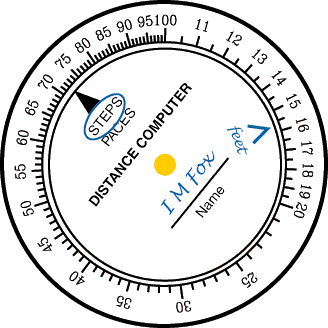# Using the Distance Computer

#### To calculate a distanced walked off, just rotate the computer so the STEPS marker points to the number of steps you counted.  Then read the distance pointed to by the "feet" marker.

• Suppose that we stepped off a distance and counted 72 steps.  Rotate the computer so STEPS points to 72.  Now "feet" points a bit above the mark that is between 15 and 16.  This mark represents 15.5, so we can call it about 15.7, or 157 feet.  (Again, 15 feet is much too short a distance.  It has to be around 150.)

•• Now, suppose that we want to step off a distance of 250 feet.  To find the number of steps this will take, rotate the computer so that the "feet" marker points to 25.  Now the STEPS marker points to 11.5, so we know that we have to go 115 steps.

•